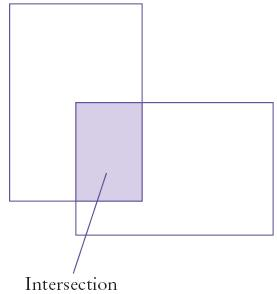The intersection method computes the intersection of two rectangles—that is, the rectangle that would be formed by two overlapping rectangles if they were drawn:You call this method as follows:

Rectangle r3 = r1.intersection(r2);

Write a program IntersectionPrinter that constructs two rectangle objects, prints them as described in Exercise P2.3, and then prints the rectangle object that describes the intersection. Then the program should print the result of the intersection method when the rectangles do not overlap. Add a comment to your program that explains how you can tell whether the resulting rectangle is empty.

Complete the following file: